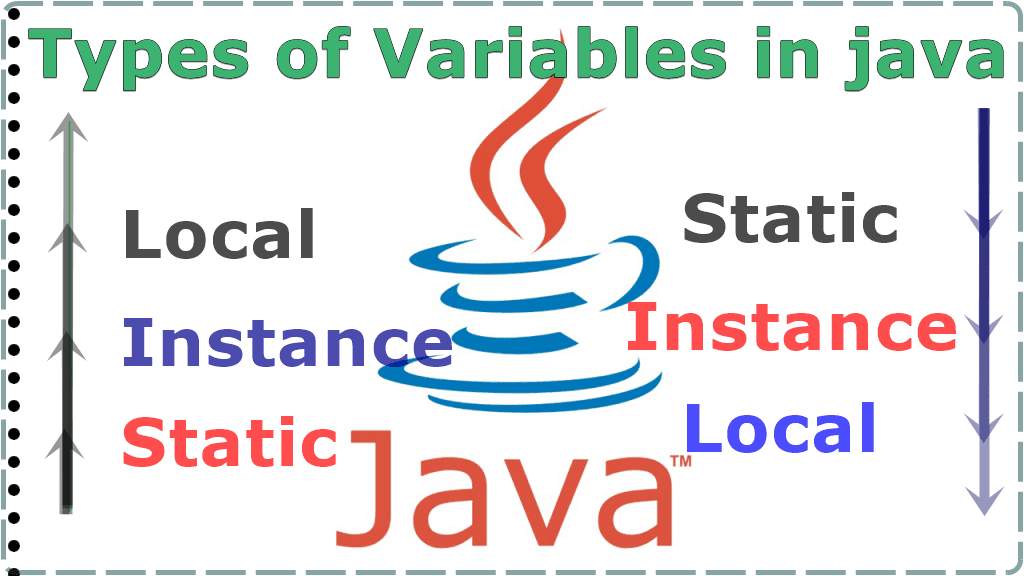# Different Types of Variables in Java Programming Language

In this post, we will talk about Different Types of Variables in the Java Programming Language. Types of Variables in java with complete explanations and examples. Like a different type of variable how to use it?. how to access it etc?. So java is the class base language the class contains five elements. Java Class Elements are Variables, Methods, Constructors, Instance Blocks and Static Blocks.

So C/C++ language contains variable int a=10 b=20 etc. in the same way Java also contains some variable parts. So to work with employee projects we need the employee id, name, salary etc. So every project required variable concepts. So before going to the variable concept. First of all, we must know what is the purpose of the variable in Java.

So the variable is used to store the values by using these values we achieve the project requirements. For example, for an employee project, we need the employee's salary, name, id etc. So in a single line variables are used to store value and by using these values we achieve the project requirements. generally, variables are 3 types.## What Are the Three Different Types of Variables in Java

1. Local Variables
2. Instance Variables
3. Static Variables

### 1. Local Variables

The variable is declared inside the method or constructor or blocks also. Those variables are called local variables

Local Variable Example

``````class {

public static void main(String[] args) {
//local variables
int a = 50;
int b = 20
System.out.println(a);
System.out.println(a);
}
}``````

The scope of the local variables is only within the method or within the constructor or within the blocks only. So simply can say that the local variables cannot be accessed outside the method, block, or constructors. If we try to access the local variable outside it generates the error message.

Example

``````class demo {

public static void main(String[] args) {
void method() {
int a = 30;
System.out.println(a); //possible
}

void method2() {
System.out.println(a); // not possible to print inside the method
}
}
}``````

Local variable Memory Allocated when the method starts and whenever the method is completed memory is released. Local variable stored in stack memory.

### 2. Instance Variables

So before the brief of instance variables, we must know areas of java language.java contains 2 types of areas, instance area, and static area. So once we understand these 2 areas then the java variables concept is very easy. So the body of the instance method is called the instance area, and the body of the static method is called the static method.

Instance Variables Example

``````class demo {
void method() // instance method
{
//instance area
}

static void method2() //static method
{
//static area
}

public static void main(String[] args) {}
}``````

• The variable that is declared inside the class but outside the method, Those variables are called instance variables.
• The scope of the instance variable is inside the class all methods, constructors and blocks can access.
• For instance, variable memory is allocated when the object is created and memory is destroyed when the object is destroyed.
• Instance variable stored in heap memory

Note: When the same area we can access the instance variable directly. But in a different area, first of all, we must allocate memory of instance variable So how memory is allocated, linked with the object, means create an object of that class in a single line, first of all, create an object of that class. And access that class instance variable and method by using that object.

Instance var, method==========>direct access========>instance area

Instance var, method==============>throw object========>static area

Example

``````class demo {
//instance var
int x = 20;
int y = 30;
void m() //instance method
{
//instance area
System.out.println(x + y);
}

public static void main(String[] args) //main method (static method)
{
//static area
demo d new demo();
System.out.println(d.x);
System.out.println(d.y);
d.m();
}
}``````

### 3. Static Variable

The variable that is declared inside the class but outside the method with a static modifier, Those variables are called static variables.

Static Variable Example

``````class {
//static variables
static int a;
static float b;
static char c;
}``````

• Static variable memory is allocated when the .class file is loading.
• The scope of the static variable is inside the class
• Static variable stored in non-heap memory.

Note:- access the static variable and method by using the class name. Reason static variable memory allocated when the .class file is loading. means before the main method load, static variable memory is allocated. Check this Java Program for Inter Thread Communication.

Example

``````class demo {
//static var
static int x = 20;
static int y = 30;
void m() //instance method
{
//instance area
System.out.println(demo.x);
System.out.println(demo.y);
}

public static void main(String[] args) //main method (static method)
{
//static area
demo d = new demo();
System.out.println(demo.x);
System.out.println(demo.y);
d.m();
}``````

Read: Different Types of Inheritance in Java With Example
Also Read: How to Achieve Abstraction in Java With Example
Previous Post
Next Post

#### post written by: Ghanendra Yadav

Hi, I’m Ghanendra Yadav, SEO Expert, Professional Blogger, Programmer, and UI Developer. Get a Solution of More Than 500+ Programming Problems, and Practice All Programs in C, C++, and Java Languages. Get a Competitive Website Solution also Ie. Hackerrank Solutions and Geeksforgeeks Solutions. If You Are Interested to Learn a C Programming Language and You Don't Have Experience in Any Programming, You Should Start with a C Programming Language, Read: List of Format Specifiers in C.
1.2.